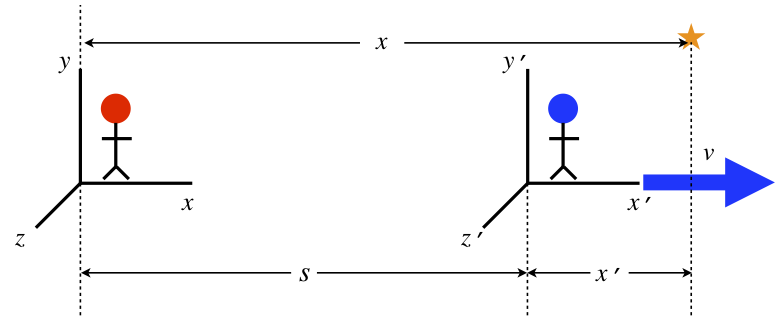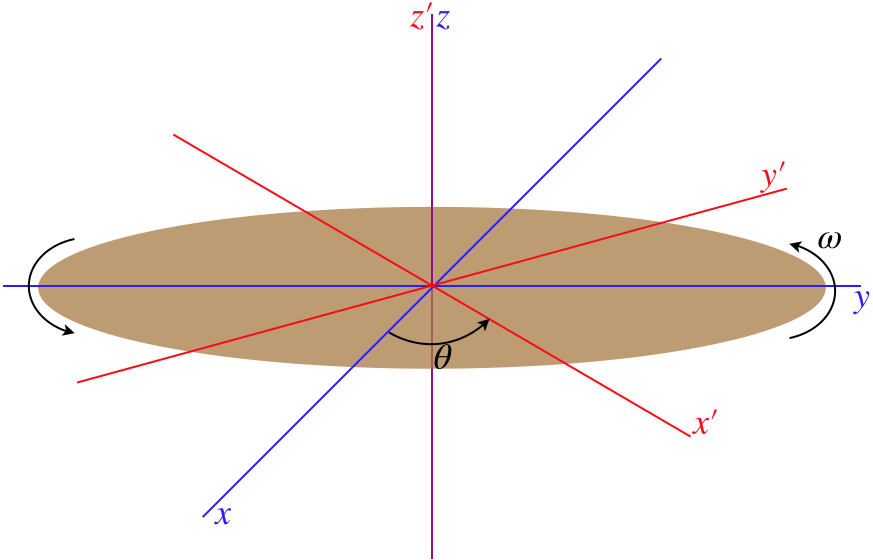$$\require{cancel}$$

# 5.2: Non-Inertial Frames

## Linear Accelerated Frames

Let us return for a moment all the way back to Section 1.8, where we discussed relative motion, and in particular, the Galilean transformation equations. If you recall, this discussion was about comparing what one observer sees to what another observer sees, and the model we used simplified things by having the observers in linear relative motion at a constant speed along their common $$x$$-axis, with their clocks synchronized so that $$t'=t=0$$ when their origins coincide:

Figure 5.2.1 – Relating Coordinates of Linear Reference Frames$\begin{array}{l} t' = t \\ x' = x-vt \\y'=y \\ z'=z \end{array}$

These equations relate how one observer logs an event in terms of position and time to how the other person logs it. Suppose these two people are observing the motion of an object. Example 1.8.2 in that section shows how we can use the transformation equations to relate the velocities witnessed by the two observers of a common object. Suppose now we want to relate the accelerations witnessed by both. Clearly with $$v$$ being a constant, its derivative is zero, which means that both observers agree upon the acceleration, even the component along the $$x$$-direction:

$\dfrac{dx'}{dt'}=\dfrac{d\left(x-vt\right)}{dt'}=\dfrac{d\left(x-vt\right)}{dt}=\dfrac{dx}{dt}-v\;\;\;\Rightarrow\;\;\; \dfrac{d^2x'}{dt'^2}=\dfrac{d^2x}{dt^2}-\cancelto{0}{\dfrac{dv}{dt}}$

Suppose, however, that the primed frame is accelerating relative to the unprimed frame. Then the separation of the frames (shown as an $$s$$ in the diagram above) has a different time dependence, and we get different transformation equations. We once again simplify things again as much as possible by insisting that:

• the acceleration is a constant value $$a$$, along the line of motion in the $$+x$$-direction
• the relative velocities of the frames at the common starting time of $$t'=t=0$$ is $$v_o$$ in the $$+x$$-direction
• the origins coincide at the common starting time of $$t'=t=0$$

This gives us the following transformation equations:

$\begin{array}{l} t' = t \\ x' = x-v_ot-\frac{1}{2}at^2 \\y'=y \\ z'=z \end{array}$

In this case, the two observers would not agree on the acceleration of the commonly-observed object, as it would differ in its $$x$$-component by an amount $$a$$:

$\dfrac{d^2x'}{dt'^2} = \dfrac{d^2x}{dt^2}-\cancelto{0}{\dfrac{dv_o}{dt}}-a$

## Fictitious Forces

Now that we are talking about accelerations, the question of forces comes up. Consider an object that remains at rest in the unprimed frame. That observer must conclude, according to Newton's second law, that it is not experiencing a net force. Naturally this observer can do a careful analysis of all the forces (friction, normal, spring, etc.) that appear on the free-body diagram for that object to confirm that the forces all add up to zero.

What about the other observer's analysis of Newton's second law? The primed observer does not see the object experiencing zero acceleration, as the transformation between their frame and the frame of the other observer demonstrates. This observer therefore concludes that there must be a net force on the object, to accelerate it. But the analysis performed by the unprimed observer who looked at all the physical forces will not come out different for the primed observer – the spring scale used to measure a normal force will not read something different for these two people. So how does this primed observer confirm Newton's second law? They must invent a new, "mystery force" that has no apparent source. We call such a force that comes about purely because the frame in which it is observed is accelerating fictitious. A frame in which there are no fictitious forces present is called an inertial frame, and frames that give rise to fictitious forces are called non-inertial.

One of the defining characteristics of a fictitious force is that it results in the same acceleration regardless of any physical features of the object, because it is the acceleration of the non-inertial frame that is actually witnessed. Given that the same acceleration is observed for all objects of all masses, the accelerated observer would have to conclude that the fictitious force is proportional to the mass of the object.

$\left. \begin{array}{l} \text{fictitious force }\mathcal F_1\text{ on }m_1\text{ and Newton's 2nd law:} && a = \dfrac{\mathcal F_1}{m_1} \\ \text{fictitious force }\mathcal F_2\text{ on }m_2\text{ and Newton's 2nd law:} && a = \dfrac{\mathcal F_2}{m_2} \end{array} \right\} \;\;\;\Rightarrow\;\;\; \dfrac{\mathcal F_1}{m_1} = \dfrac{\mathcal F_2}{m_2}$

But wait, there is a force we use all the time that satisfies this very property – gravity! The force of gravity is given by $$mg$$, and indeed every object in free fall is seen to accelerate exactly the same amount. So is gravity a fictitious force? We know there is a cause for this force – the earth exerts the force on all objects in proportion to their mass, so maybe gravity just coincidentally resembles a fictitious force. But in fact Einstein, in his theory of general relativity, proposed that gravity is essentially a fictitious force, in his general theory of relativity. He posited what he called the equivalence principle, which stated that an observer in a closed room in the absence of gravity that is accelerating in the "upward" direction at a rate of $$9.8\frac{m}{s^2}$$ can perform no experiment that will distinguish between that circumstance and the case of the identical closed room on the surface of the earth.

Figure 5.2.2 – Equivalence PrincipleAs simple as this equivalence might seem to be, it is nevertheless very complicated mathematically to relate a force that attracts objects toward a single point (the center of the planet) to the fictitious force seen in a linearly-accelerated frame, so we unfortunately have to end our discussion of general relativity here and leave it for future coursework in physics.

## Rotating Frames

As we know, acceleration that involves speeding up or slowing down in a straight line is not the only variety. We now want to look at how to transform between an inertial frame and one that is rotating around a fixed point in the inertial frame. We will place the origin of the inertial frame on the axis of rotation of the rotating frame, with the axis perpendicular to the plane of rotation coinciding with the $$z$$-axis of the inertial frame. The red axes in the figure below are rotating at a constant rate while the blue axes remain stationary.

Figure 5.2.3 – Relating Coordinates of Rotational Reference FramesLet's imagine an object at rest on the positive $$x'$$-axis at the moment when all three axes are aligned. The observer in the unprimed frame that is watching this object will see this object moving in a circle, and at the moment the axes are aligned, it is moving in the $$+y$$-direction. If we call the distance from the $$z$$-axis $$r$$, then the speed of the object as seen by the observer in the unprimed frame is $$\omega r$$. Putting the magnitude and direction together gives us the velocity vector $$\omega r\; \widehat j$$ for this object, as measured by the unprimed observer.

We can write this velocity in terms of the position vector $$\overrightarrow r = r\;\widehat i$$ and the rotation vector (which we get from the right-hand rule) $$\overrightarrow \omega = \omega\;\widehat k$$ as follows:

$\omega r\; \widehat j = \overrightarrow \omega \times \overrightarrow r$

This follows very simply, because the cross product of $$\widehat k \times \widehat i$$ is just $$\widehat j$$.

The nice thing about this way of expressing the velocity is that it doesn't depend upon the specific circumstance of being on the $$x$$-axis. Wherever the object happens to be, this cross-product combination of the angular velocity vector and the position vector gives the proper magnitude and direction of the velocity vector seen by the unprimed frame.

If an object is instead at rest in the unprimed frame, then the observer in the primed frame will see the same thing, but in the opposite direction: $$-\overrightarrow \omega \times \overrightarrow r$$. Now suppose the object happens to be moving in the unprimed frame. To get the velocity vector seen in the primed frame, we simply add vectors according to Equation 1.8.3:

$\overrightarrow v_{object\;rel\;to\;primed} = \overrightarrow v_{object\;rel\;to\;unprimed} + \overrightarrow v_{unprimed\;rel\;to\;primed} \;\;\; \Rightarrow \;\;\; \overrightarrow v' = \overrightarrow v - \overrightarrow \omega \times \overrightarrow r$

This is the rotational version of the Galilean transformation equations. If we write the velocities in terms of the derivatives of position vectors, we get an interesting (and useful) form of this relation:

$\dfrac{d}{dt}\overrightarrow r' = \dfrac{d}{dt}\overrightarrow r -\overrightarrow \omega \times \overrightarrow r \;\;\;\Rightarrow\;\;\; \left[\dfrac{d}{dt}\right]_{in\;primed\;frame} = \left[\dfrac{d}{dt}\right]_{in\;unprimed\;frame} -\overrightarrow \omega \times$

This gives us a translation between derivatives in the two frames - any derivative of a vector in the primed frame is equivalent to a derivative of the vector in the unprimed frame minus the cross product of the rotation vector with that same vector.

## More Fictitious Forces

Every point on the rotating frame is accelerating, so if we are observing from that frame, we should expect to witness mysterious accelerations of objects that are not accelerating in the unprimed frame. That is, the primed observer will not be able to pinpoint a true physical force causing it to accelerate, and will need to invent a fictitious force to explain this acceleration with Newton's second law. To determine this force, we first need to derive a translation between accelerations in the two frames. We get the acceleration by taking the derivative of the velocity, so we apply Equation 5.28 to Equation 5.27: